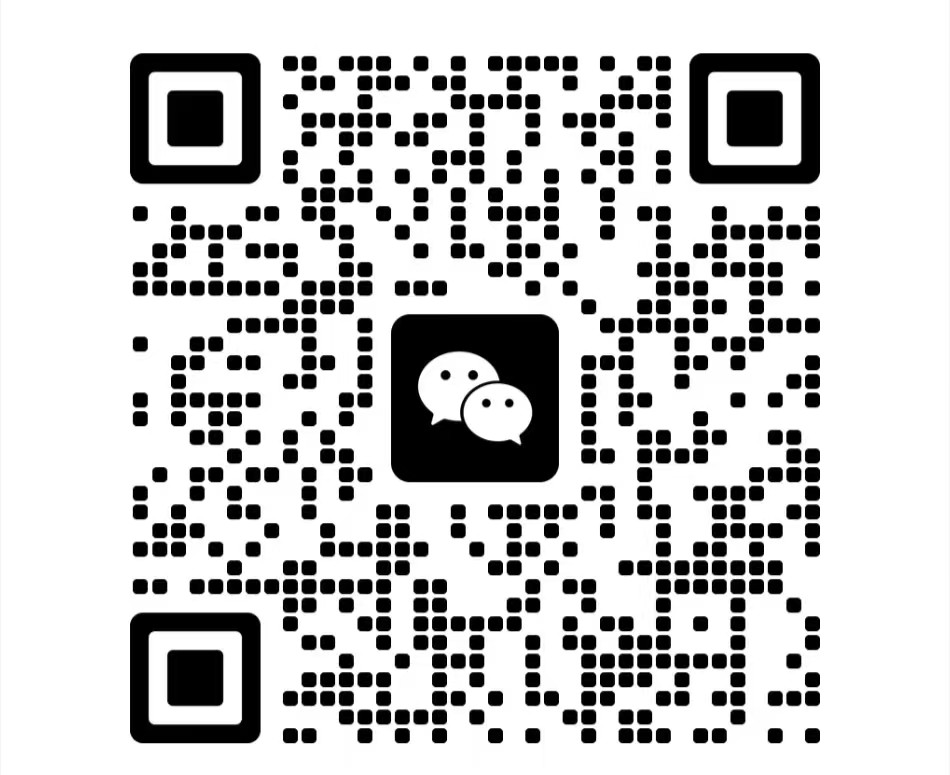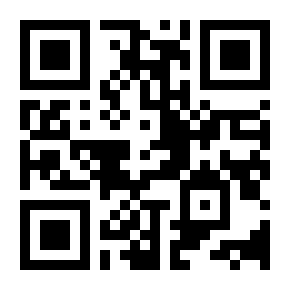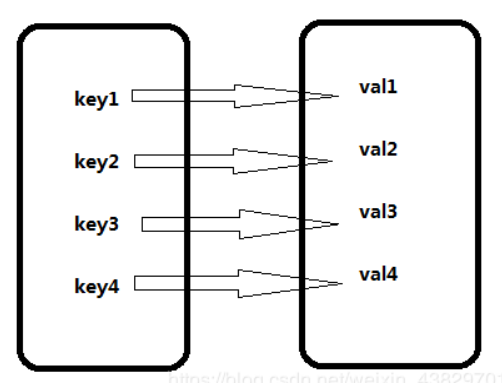# Python dict字典知识

2021-05-16 新闻来源：网淘巴 围观:308
''

Python 字典（dict）是一种无序的、可变的序列，它的元素以“键值对（key-value）”的形式存储。相对地，列表（list）和元组（tuple）都是有序的序列，它们的元素在底层是挨着存放的。Python 中的字典类型相当于 Java 或者 C++ 中的 Map 对象。

>>> a = {'one': 1, 'two': 2, 'three': 3}  #a是一个字典类型
>>> type(a)
<class 'dict'>

## Python创建字典

#### 1) 使用 { } 创建字典

dictname = {'key':'value1', 'key2':'value2', ..., 'keyn':valuen}

1. #使用字符串作为key
2. scores = {'数学': 95, '英语': 92, '语文': 84}
3. print(scores)
4.
5. #使用元组和数字作为key
6. dict1 = {(20, 30): 'great', 30: [1,2,3]}
7. print(dict1)
8.
9. #创建空元组
10. dict2 = {}
11. print(dict2)

{'数学': 95, '英语': 92, '语文': 84}
{(20, 30): 'great', 30: [1, 2, 3]}
{}

#### 2) 通过 fromkeys() 方法创建字典

Python 中，还可以使用 dict 字典类型提供的 fromkeys() 方法创建带有默认值的字典，具体格式为：

dictname = dict.fromkeys(list，value=None)

1. knowledge = ['语文', '数学', '英语']
2. scores = dict.fromkeys(knowledge, 60)
3. print(scores)

{'语文': 60, '英语': 60, '数学': 60}

#### 3) 通过 dict() 映射函数创建字典

a = dict(str1=value1, str2=value2, str3=value3)str 表示字符串类型的键，value 表示键对应的值。使用此方式创建字典时，字符串不能带引号。
#方式1
demo = [('two',2), ('one',1), ('three',3)]
#方式2
demo = [['two',2], ['one',1], ['three',3]]
#方式3
demo = (('two',2), ('one',1), ('three',3))
#方式4
demo = (['two',2], ['one',1], ['three',3])
a = dict(demo)

keys = ['one', 'two', 'three'] #还可以是字符串或元组
values = [1, 2, 3] #还可以是字符串或元组
a = dict( zip(keys, values) )

1. # 创建空的字典
2. d = dict()
3. print(d)

{}

## Python 访问字典

Python 访问字典元素的具体格式为：

dictname[key]

1. tup = (['two',26], ['one',88], ['three',100], ['four',-59])
2. dic = dict(tup)
3. print(dic['one']) #键存在
4. print(dic['five']) #键不存在

88
Traceback (most recent call last):
File "C:\Users\mozhiyan\Desktop\demo.py", line 4, in <module>
print(dic['five'])  #键不存在
KeyError: 'five'

get() 方法的语法格式为：

dictname.get(key[,default])

get() 使用示例：

1. a = dict(two=0.65, one=88, three=100, four=-59)
2. print( a.get('one') )

88

1. a = dict(two=0.65, one=88, three=100, four=-59)
2. print( a.get('five', '该键不存在') )

## Python删除字典

1. a = dict(two=0.65, one=88, three=100, four=-59)
2. print(a)
3. del a
4. print(a)

{'two': 0.65, 'one': 88, 'three': 100, 'four': -59}
Traceback (most recent call last):
File "C:\Users\mozhiyan\Desktop\demo.py", line 4, in <module>
print(a)
NameError: name 'a' is not defined

Python 自带垃圾回收功能，会自动销毁不用的字典，所以一般不需要通过 del 来手动删除。

`一、网淘巴提供的资源部分来自互联网，如果有侵犯您的版权或其他权益，请说明详细缘由并提供版权或权益证明然后发送到邮箱：jjq_hn@wtao8.com ，我们会在看到邮件的第一时间内为您处理。二、网友通过wtao8.com途径发表的一切信息，本站有权在本网站范围内引用、发布、转载用户在本站发布的内容。本站对于用户发布的内容所引发的版权、署名权的异议、纠纷不承担任何责任。传统媒体转载须事先与原作者和本站联系。提交者发言纯属个人行为，与本网站立场无关。`